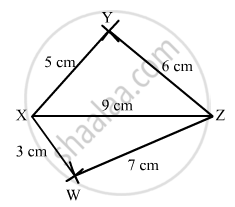Share

# Construct a Quadrilateral Xyzw in Which Xy = 5 Cm, Yz = 6 Cm, Zw = 7 Cm, Wx = 3 Cm and Xz = 9 Cm. - Mathematics

Course
ConceptConstructing a Quadrilateral When Two Diagonals and Three Sides Are Given

#### Question

Construct a quadrilateral XYZW in which XY = 5 cm, YZ = 6 cm, ZW = 7 cm, WX = 3 cm and XZ = 9 cm.

#### Solution

$\text { Steps of construction: }$

$\text { Step I: Draw XZ = 9 cm }$

$\text { Step II: With X as the centre and radius 5 cm, draw an arc above XZ } .$

$\text { Step III: With Z as the centre and radius 6 cm, draw an arc to intersect the arc drawn in Step II at Y above XZ }.$

$\text { Step IV: With Z as the centre and radius 7 cm, draw an arc below XZ .}$

$\text { Step V: With X as the centre and radius 3 cm, draw an arc to intersect the arc drawn in Step IV at W below XZ }.$

$\text { Step VI: Join XY, YZ, ZW and XW } .$

$\text { The quadrilateral WXYZ so obtained is the required quadrilateral } .$Is there an error in this question or solution?

#### APPEARS IN

RD Sharma Solution for Mathematics for Class 8 by R D Sharma (2019-2020 Session) (2017 to Current)
Chapter 18: Practical Geometry (Constructions)
Ex. 18.1 | Q: 3 | Page no. 4
Solution Construct a Quadrilateral Xyzw in Which Xy = 5 Cm, Yz = 6 Cm, Zw = 7 Cm, Wx = 3 Cm and Xz = 9 Cm. Concept: Constructing a Quadrilateral - When Two Diagonals and Three Sides Are Given.
S